### Working with formulas

Using Quattro Pro, you can create math formulas. The following characters are used when creating a formula:

 Character Result . establishes the following data as a value + establishes the following data as a positive value - establishes the following data as a negative value ( ) establishes the following data as a formula and calculates first anything contained in the parentheses @ establishes the following data as a function # establishes the following data as a logical equation \$ establishes the following data as a currency value = establishes the following data as a value

As well, a formula can use any of the following arguments:

 Argument Examples Numbers 1948, -84, 43.23, 12½ Cell coordinates B12, G29..G31, B:A3..D6, or [NOTEBK2]A:A1 Cell names EXPENSES (which may reference D5..D10) Spreadsheet functions @SUM(B1..B24) Text set off by ” “ “PROFIT” or “Dear Mr.”

You create formulas using cell data, such as column totals.

You can also create text formulas. Text formulas are any formulas that have a textual result.

Quattro Pro also allows you to create logical formulas that evaluate true or false. For example, the result of the formula +C3<10 displays either 1 or 0 depending on the value of cell C3.

Displaying error sources directly in your cells shows you the cause of any problem with your formula. As well, you can convert formulas to values; the value appears in the cell and the input line, replacing the formula.

For additional information about creating formulas, see “Operator precedence”, “Guidelines for solving errors in formulas”, and “Working with arrays”.

#### To create a math formula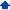1 Type a plus sign (+) in a blank cell.
 2 Type a formula.
 3 Press Enter.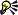• To view formula results as you enter the formula data, press F9.

#### To create a formula from cell data1 Type a plus sign (+) in a blank cell where you want to enter the formula.
 2 Begin typing the formula
 3 Click the cell that contains the data to insert in the formula.
 4 Finish typing the formula.If the cell containing the data has a cell name, you can type the name into the formula instead of clicking on the cell. Make sure the insertion point for the name is to the right of an operator or opening parenthesis in the formula.

#### To create a text formula1 Type a plus sign (+) in a blank cell.
 2 Type the formula using an ampersand (&) to combine text strings.
 For example,+C4&”Review” enters the text in cell C4, then the word Review.
 3 Press Enter.• If you want to add a space between strings, type it inside the quotation marks. For example,+C4&” Review” enters the text in cell C4, a space, then the word Review.

#### To create a formula that evaluates to true or false1 Click a blank cell.
 2 Type a formula using one of the logical operators.
 3 Press Enter.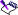• If the statement is true, a 1 displays in the cell. If the statement is false, a 0 displays.
 • For an explanation of operators, see “Operator precedence.”

#### To display error sources in cells1 Click Format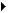Notebook properties.
 2 Click the Recalc settings tab.
 3 Enable the Audit errors check box.• When you enable the Audit errors check box, each problem cell displays the sheet and cell reference where the problem started. The notebook name is included if the problem cell is in another notebook.

#### To convert a formula to a value1 Select a cell containing a formula.
 2 Click EditConvert to values.
 3 Type the destination cells in the To box.• To replace a formula in a cell with its value, specify the same cells in the To box as are in the From box.
 • &nbs p; To copy the value of a formula to another part of the notebook, specify the top-left cell to which you want to copy the value.• You can also specify the addresses of the destination cells by clicking the Range picker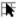to the right of the relevant box and selecting a cell.